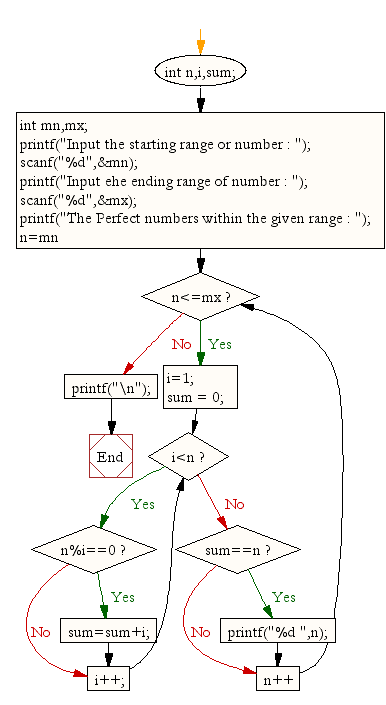﻿ C : Find perfect numbers within a given number of range# C Exercises: Find perfect numbers within a given number of range

## C For Loop: Exercise-28 with Solution

Write a c program to find the perfect numbers within a given number of range.

Sample Solution:

C Code:

``````/*Perfect number is a positive number which sum of all positive divisors excluding
that number is equal to that number. For example 6 is perfect number since divisor of 6 are 1, 2 and 3.
Sum of its divisor is 1 + 2+ 3 = 6*/
#include <stdio.h>

void  main(){
int n,i,sum;
int mn,mx;
printf("Input the starting range or number : ");
scanf("%d",&mn);
printf("Input the ending range of number : ");
scanf("%d",&mx);
printf("The Perfect numbers within the given range : ");
for(n=mn;n<=mx;n++){
i=1;
sum = 0;
while(i<n){
if(n%i==0)
sum=sum+i;
i++;
}
if(sum==n)
printf("%d ",n);
}
printf("\n");
}
```
```

Sample Output:

```Input the starting range or number : 1
Input the ending range of number : 50
The Perfect numbers within the given range : 6 28
```

Flowchart:C Programming Code Editor:

Improve this sample solution and post your code through Disqus.

What is the difficulty level of this exercise?

Test your Programming skills with w3resource's quiz.

﻿

## C Programming: Tips of the Day

What is the difference between printf() and puts() in C?

puts is simpler than printf but be aware that the former automatically appends a newline. If that's not what you want, you can fputs your string to stdout or use printf.

Ref :https://bit.ly/3f2fwcH